# Example: {rn}

Previous: Squeeze Theorem Example

Next: Videos on Infinite Sequences

## Example

Determine the values of r so that the sequence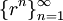is convergent.

## Complete Solution

We can solve this problem by considering cases for the value of r.

### Case 1

If r > 1, then  rn tends to infinity as n tends to infinity. The sequence is divergent in this case.

### Case 2

If r= 1, thenso the sequence is convergent for this case.

### Case 3

If, thenso the sequence is convergent for this case.

### Case 4

If, thendoes not exist, so the sequence is divergent for this case.

### Case 5

If, thentends to negative infinity asdoes not tend to a single finite number. The sequence is divergent in this case.

### Summary

Therefore, the sequenceis convergent when.

## Explanation of Each Step

### Case 1

Consider the case when. Then our sequence becomeswhich tends to infinity.

### Case 2

Here we are using a fundamental property of limits, that the limit of a constant equals that constant:for any constant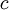and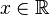.

### Case 3

Consider the case when. Then our sequence becomeswhich tends to zero.

Similarly, if. Then our sequence becomes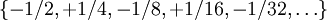which also tends to zero.

### Case 4

In this case, we have the sequenceAsapproaches infinity the sequence does not approach a unique value, so the limit does not exist.

### Case 5

This case is similar to Case 1. Consider the case when. Then our sequence becomes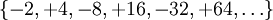The terms alternate between positive and negative numbers, and do not tend to a single finite number.

## Possible Challenge Areas

### Connecting Results to Definition of Convergence

In each of the cases, we used a limit to determine whether the sequence is convergent. According to our definition of convergence of a sequence, as long as our respective limits exist, then the sequence converges.

Previous: Squeeze Theorem Example

Next: Videos on Infinite Sequences